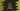# 4 different C# ways to find the LCM of two numbers## C# program to find the LCM of two numbers:

In this post, we will learn how to find the LCM of two numbers in C#. The LCM or least common multiple of two numbers is equal to the smallest number that is multiple of both the numbers.

For example, the LCM of 2 and 5 is 10.

The first multiples of 2 are 2, 4, 6, 8, 10, 12, 14, 16, 18… and the first multiples of 5 are 5, 10, 15, 20… etc. So, the lowest common multiple or least common multiple is 10.

### Method 1: C# program to find the LCM of two numbers by using a for loop:

In this program, we will use a for loop to find the LCM of two numbers. The program will use the below algorithm:

• Read the numbers as inputs from the user.
• Find the larger number.
• Run a loop starting from the larger number. On each iteration, increment the iterator value by larger number. If the iterator value is divisible by both numbers, it is the required LCM.

Below is the complete C# program that finds the LCM of two user input numbers:

``````namespace Program
{

class Program
{
static void Main(string[] args)
{
int i, First, Second, Larger, LCM;

Console.WriteLine("Enter the first number:");

Console.WriteLine("Enter the second number:");

Larger = First > Second ? First : Second;

for (i = Larger; ; i += Larger)
{
if (i % First == 0 && i % Second == 0)
{
LCM = i;
break;
}
}

Console.WriteLine("LCM: " + LCM);
}
}
}``````

Here,

• i, First, Second, Larger and LCM are integer variables.
• The user input first and the second numbers are read and stored in the variables First and Second.
• Find the larger number and store it in the Larger variable.
• Run the for loop from i = Larger. On each iteration, the value of i is incremented by Larger.
• If i is divisible by First and Second, i is the LCM. Exit from the loop if the LCM is found.
• At the end of the loop, print the value of LCM.

If you run this program, it will print output as like below:

``````Enter the first number:
2
Enter the first number:
3
LCM: 6

Enter the first number:
2
Enter the first number:
10
LCM: 10``````

### Method 2: C# program to find the LCM of two numbers by using a while loop:

We can also use a while loop to find the LCM of two numbers in a similar way. Let me change the above program to use while loop instead of for loop:

``````namespace Program
{

class Program
{
static void Main(string[] args)
{
int First, Second, Larger, LCM;

Console.WriteLine("Enter the first number:");

Console.WriteLine("Enter the second number:");

Larger = First > Second ? First : Second;

LCM = Larger;
while (!(LCM % First == 0 && LCM % Second == 0))
{
LCM += Larger;
}

Console.WriteLine("LCM: " + LCM);
}
}
}``````

This is almost similar to the above program. The while loop will run until the value of LCM is divisible by both First and Second. Before the loop starts, LCM is assigned Larger. On each iteration, its value is incremented by Larger.

If you run this program, it will print similar results.

### Method 3: Recursive method to find the LCM of two numbers:

A recursive method calls itself again and again to find the result. We can use a recursive method to find the LCM of two numbers.

``````namespace Program
{

class Program
{
static int FindLCM(int LCM, int First, int Second, int Larger)
{
if (LCM % First == 0 && LCM % Second == 0)
{
return LCM;
}
return FindLCM(LCM + Larger, First, Second, Larger);
}
static void Main(string[] args)
{
int First, Second, Larger, LCM;

Console.WriteLine("Enter the first number:");

Console.WriteLine("Enter the second number:");

Larger = First > Second ? First : Second;

LCM = FindLCM(Larger, First, Second, Larger);

Console.WriteLine("LCM: " + LCM);
}
}
}``````

Here,

• FindLCM is a recursive method that finds the lcm of two numbers recursively. It takes the lcm value, first number, second number and the larger number as the parameters and returns the LCM.
• If the LCM is divisible by both first and the second numbers, it returns the LCM that is passed to this method. Else, it calls the same method FindLCM with LCM incremented by Larger.

It will give similar result.

### Method 4: By calculating the GCD:

The GCD of two numbers is the greatest common divisor or the larger divisor of these two numbers. The product of LCM and GCD of two numbers is equal to the product of the numbers. So, if we calculate the GCD, we can find out the LCM by dividing the product of the numbers by the GCD value.

``````namespace Program
{

class Program
{
static int FindGCD(int First, int Second)
{
if (Second == 0)
return First;
return FindGCD(Second, First % Second);
}
static int FindLCM(int First, int Second)
{
return (First * Second) / FindGCD(First, Second);
}

static void Main(string[] args)
{
int First, Second, LCM;

Console.WriteLine("Enter the first number:");

Console.WriteLine("Enter the second number:");